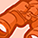Announcements
The way to achieve your own success is the willingness to help somebody else. Go for it!
cancel
Showing results for
Did you mean:Contributor II

## Rounding Values for calculation

Hi all,

i need to take average 4 weeks data, need compare with one absolute number and need to show output as Increase or decrease or Equal. When i am taking average it use running numbers, and my comparing number is with single decimal point. Can anybody help me to get numbers in one decimal point to calculation.

Eg : 1) average value : 34.5643 my absolute number is 35. so it should be calculated as 34.6<35.

2) average value : 34.9643 my absolute number is 35. so it should be calculated as 35.0=35. (in this case QV calculating as 34.96 and gives output as Decrease, but my actual output should be Equal). Thanks in advance.

Regards,

Saravanan

Labels (3)

• ### General Question

1 Solution

Accepted SolutionsMVP

Round(34.5643, 0.1) = 34.6